Question

# the differential showing the relation x (t) and y (t) Find the equation.

For the system whose block diagram is given below, the differential showing the relation x (t) and y (t)
Find the equation.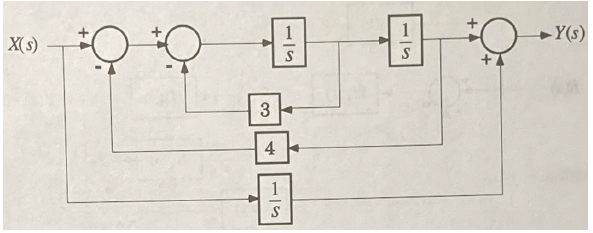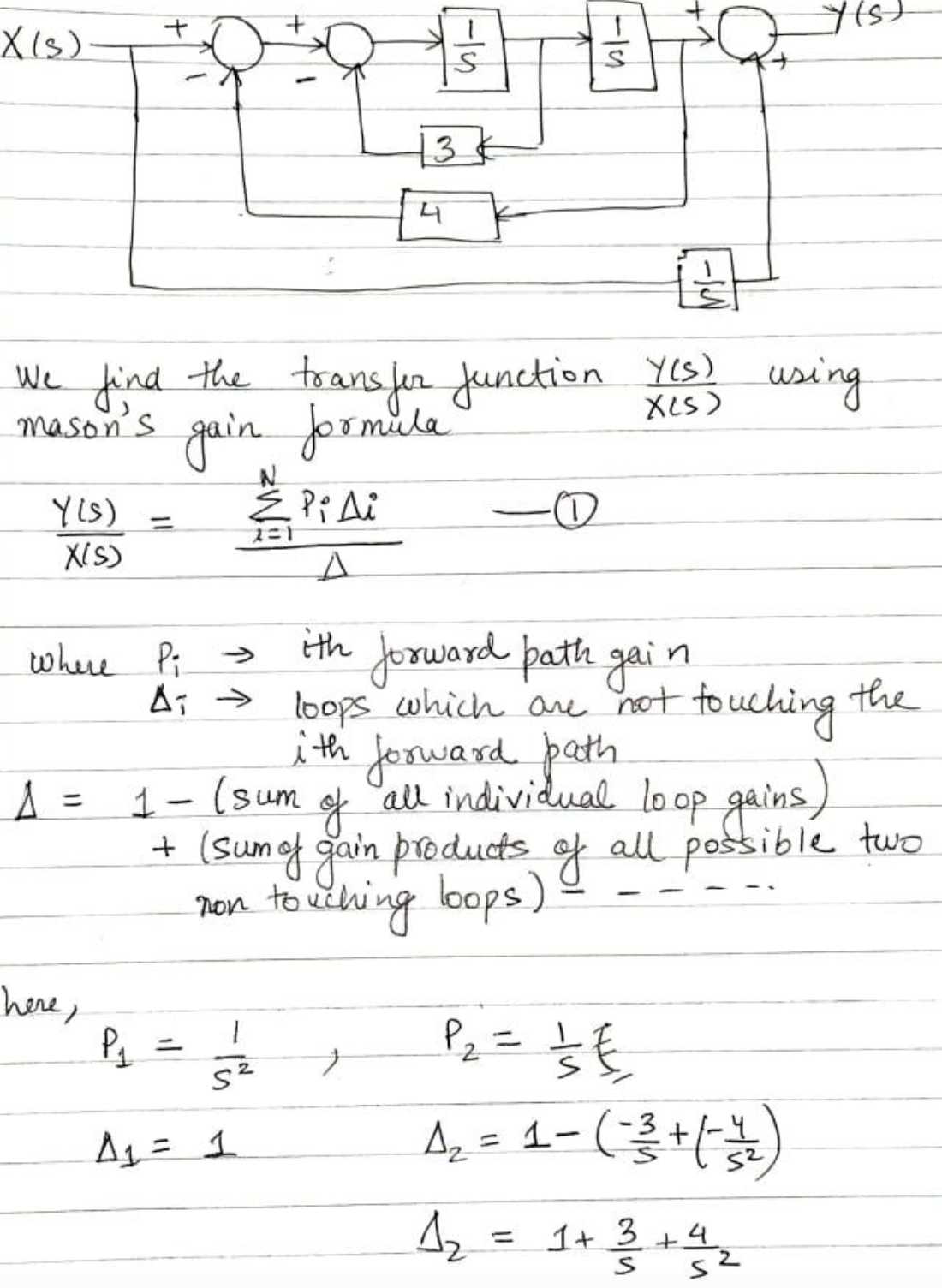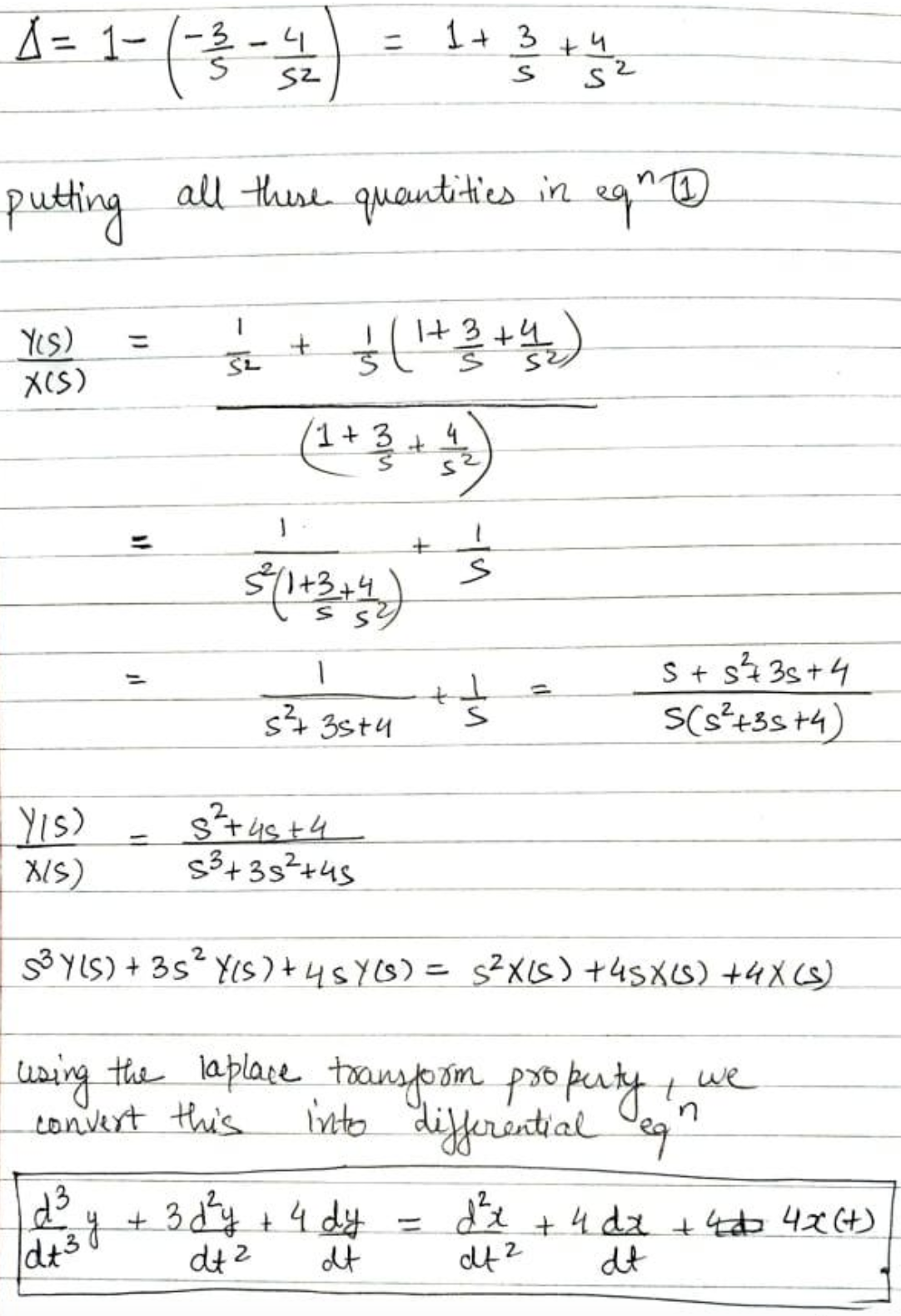#### Earn Coins

Coins can be redeemed for fabulous gifts.

Similar Homework Help Questions
• ### Find a recurrence relation for the power series solutions of differential equation y" - 2xy' +...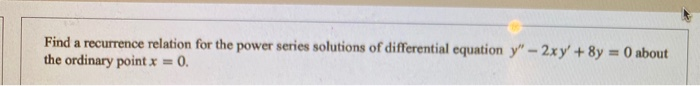Find a recurrence relation for the power series solutions of differential equation y" - 2xy' + 8y = 0 about the ordinary point x = 0.

• ### Seek power series solution of the given differential equation about the given point x0; find the recurrence relation

Seek power series solution of the given differential equation about the given point x0; find the recurrence relation.(1-x)y'' + y = 0; x0 = 0

• ### 2. Consider the causal LTI system in which the input x(t) and output y(t) are related...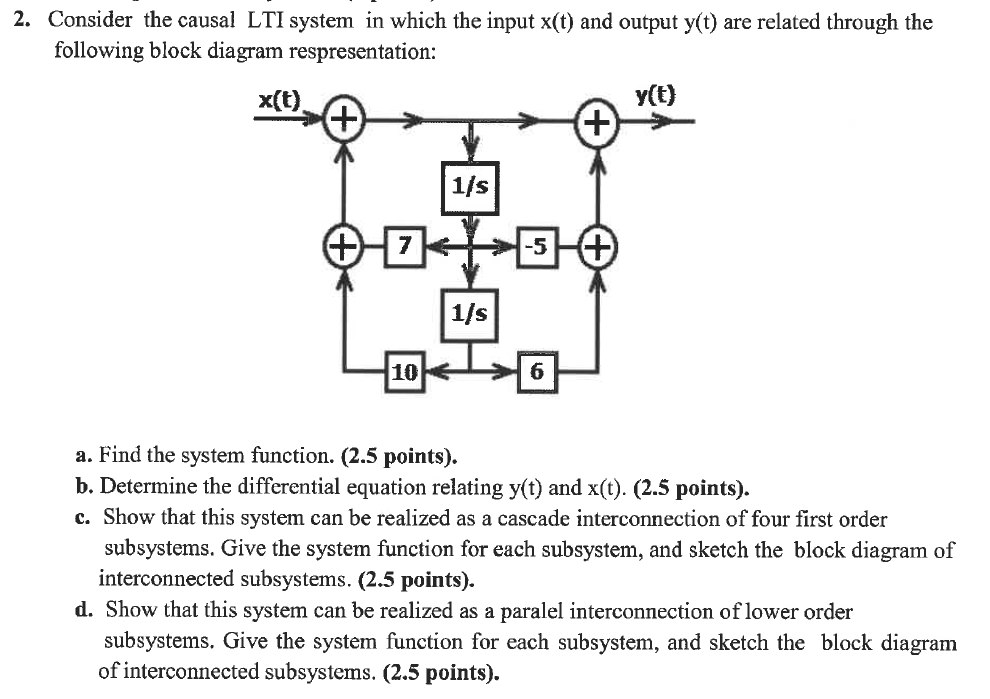2. Consider the causal LTI system in which the input x(t) and output y(t) are related through the following block diagram respresentation: x(t) y(t) + 1/s # 7 -5 1/s 10 6 a. Find the system function. (2.5 points). b. Determine the differential equation relating y(t) and x(t). (2.5 points). c. Show that this system can be realized as a cascade interconnection of four first order subsystems. Give the system function for each subsystem, and sketch the block diagram of...

• ### The input x(t) and output y(t) of a causal LTI system are related through the block-diagram...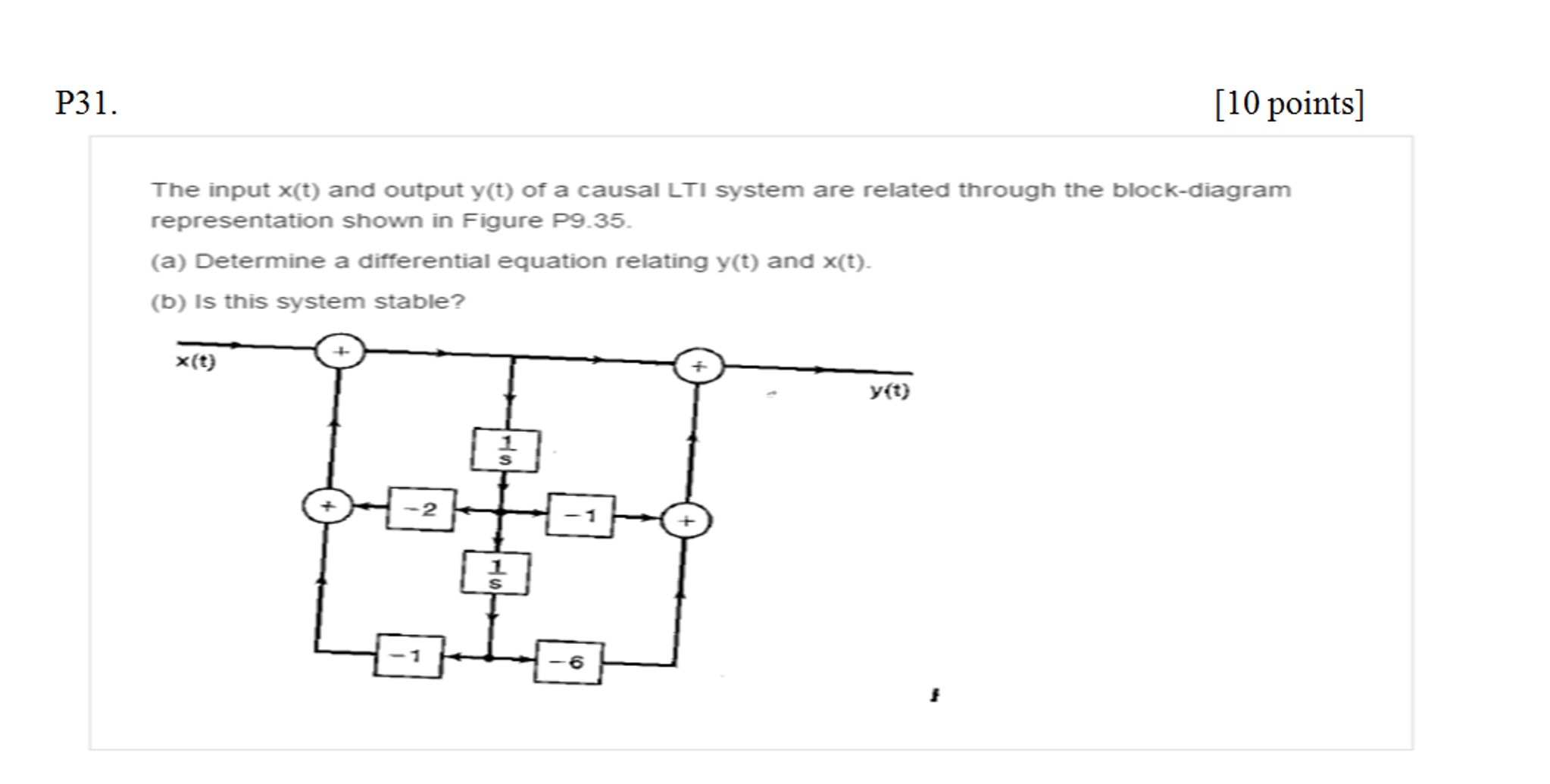The input x(t) and output y(t) of a causal LTI system are related through the block-diagram representation shown in Figure P 9.35. Determine a differential equation relating y(t) and x(t). is this system stable?

• ### Digital Signal Processing Homework #4 1. Find the solution of the differential equation: y+4y+3y = x+2x...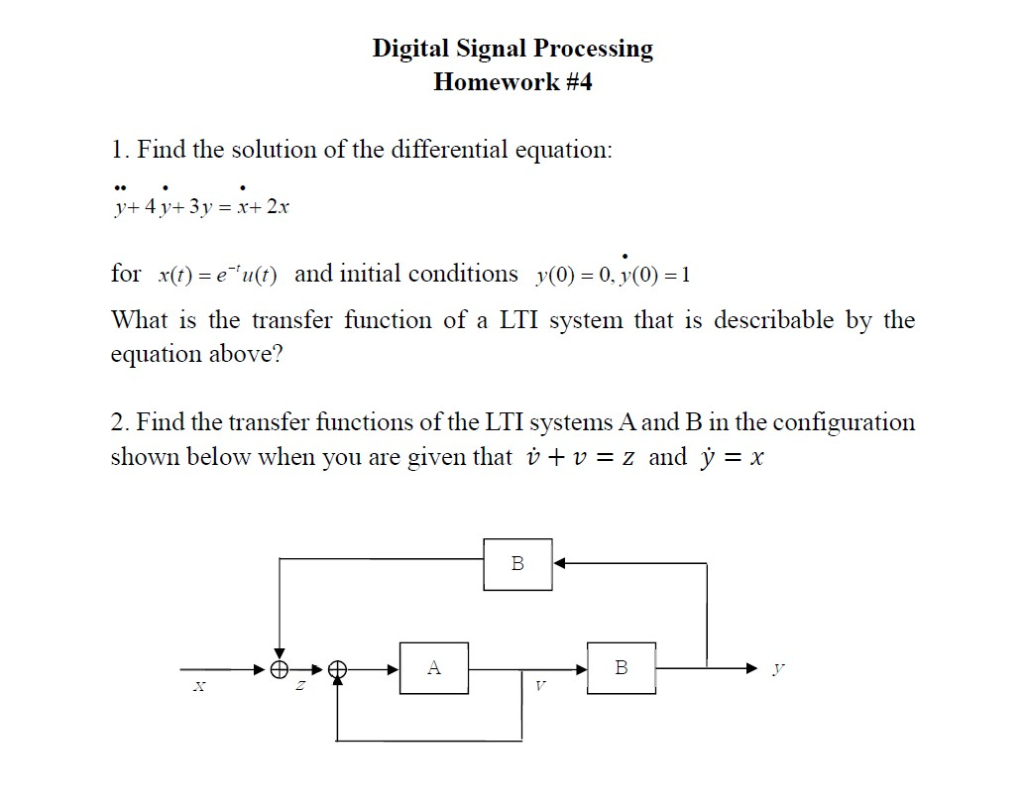Digital Signal Processing Homework #4 1. Find the solution of the differential equation: y+4y+3y = x+2x for x(t)-e'u(t) and initial conditions y(0) 0, (0) 1 What is the transfer function of a LTI system that is describable by the equation above? 2. Find the transfer functions of the LTI systems A and B in the configuration shown below when you are given that v v-z and y-x

• ### Consider the linear system given by the following differential equation y(4) + 3y(3) + 2y +...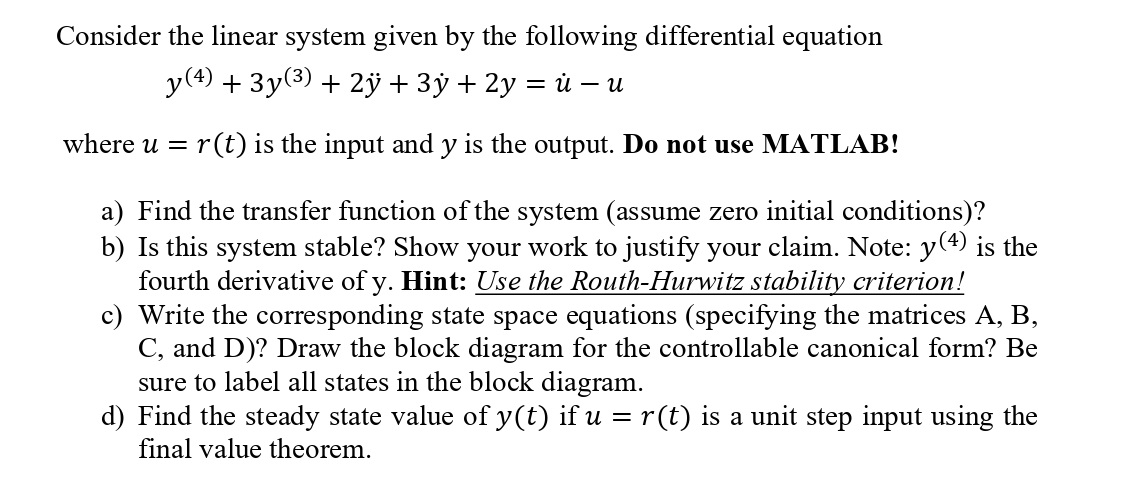Consider the linear system given by the following differential equation y(4) + 3y(3) + 2y + 3y + 2y = ů – u where u = r(t) is the input and y is the output. Do not use MATLAB! a) Find the transfer function of the system (assume zero initial conditions)? b) Is this system stable? Show your work to justify your claim. Note: y(4) is the fourth derivative of y. Hint: Use the Routh-Hurwitz stability criterion! c) Write the...

• ### Consider the differential equation, L[y] = y'' + p(t)y' + q(t)y = 0, (1) whose coefficients...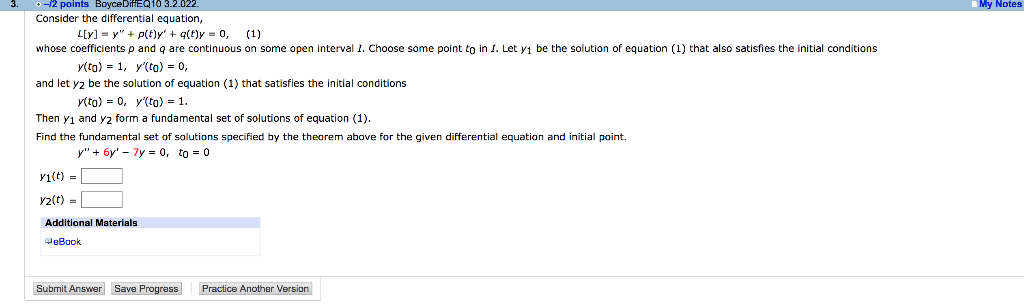Consider the differential equation, L[y] = y'' + p(t)y' + q(t)y = 0, (1) whose coefficients p and q are continuous on some open interval I. Choose some point t0 in I. Let y1 be the solution of equation (1) that also satisfies the initial conditions y(t0) = 1, y'(t0) = 0, and let y2 be the solution of equation (1) that satisfies the initial conditions y(t0) = 0, y'(t0) = 1. Then y1 and y2 form a fundamental set...

• ### Q3. Let Grepresent a system described by the following differential equation: diye) + y(t) = dre)...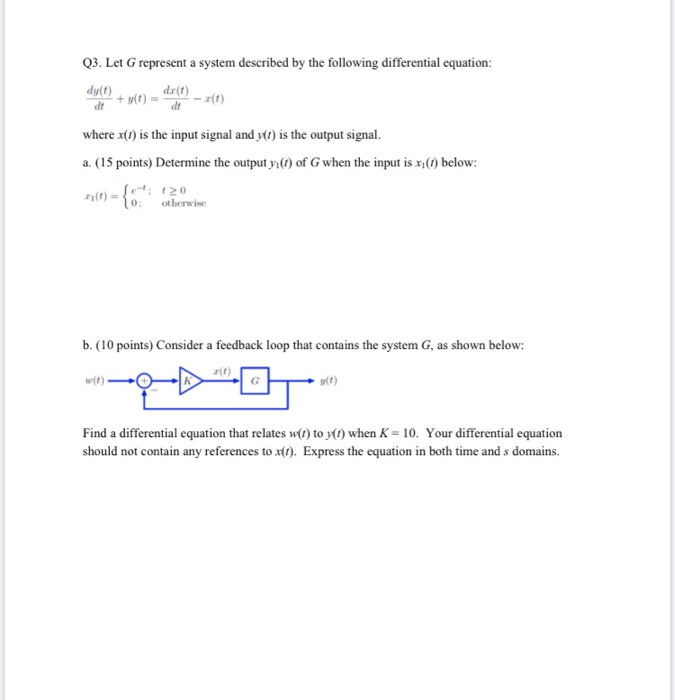Q3. Let Grepresent a system described by the following differential equation: diye) + y(t) = dre) - 10) where x(1) is the input signal and y(t) is the output signal. a. (15 points) Determine the output yı(t) of G when the input is x below: 0 0 : otherwise b. (10 points) Consider a feedback loop that contains the system G, as shown below: Find a differential equation that relates w(l) to yo) when K = 10. Your differential equation...

• ### Find the differential equation relating input x(t) to output y(t) for the following circuit: Please be...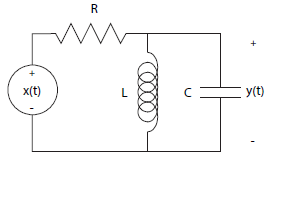Find the differential equation relating input x(t) to output y(t) for the following circuit: Please be descriptive, I would like to understand all parts of the equation better. x(t) y(il

• ### Solve the differential equation below with initial conditions. . Find the recurrence relation and compute the...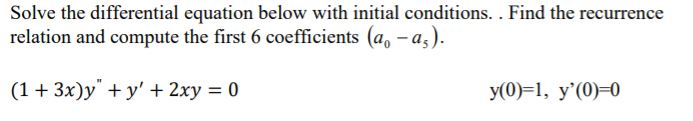Solve the differential equation below with initial conditions. . Find the recurrence relation and compute the first 6 coefficients (a -a,) (1 3x)y y' 2xy 0 y(0) 1, y'(0)-0